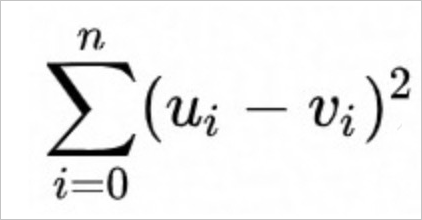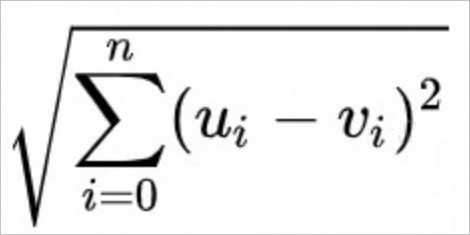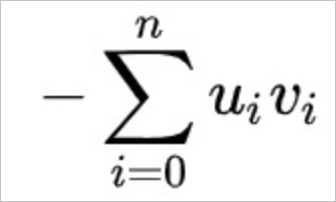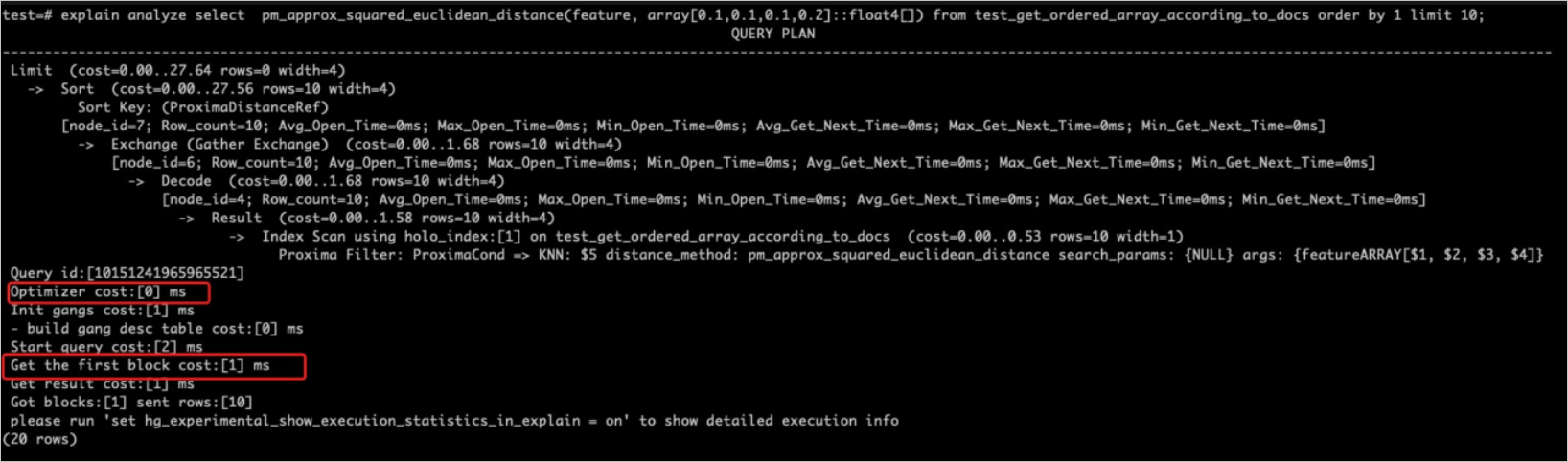This topic describes how to perform vector processing in Hologres.

## Background information

Proxima is a high-performance software library developed by Alibaba DAMO Academy. It allows you to search for the nearest neighbors of vectors. Proxima provides higher stability and performance than similar open source software such as Facebook AI Similarity Search (Fassi). Proxima provides basic modules that have leading performance and effects in the industry and allows you to search for similar images, videos, or human faces. Hologres is deeply integrated with Proxima to provide a high-performance vector search service.

## Proxima

• Terms
• Feature vector: A vector is the algebraic representation of an entity or application. The vector abstracts the relationship between entities into the distance in the vector space, and the distance indicates the degree of similarity. Examples: height, age, gender, and region.
• Vector search: fast search and match performed on a feature vector dataset. K-nearest neighbors (KNN) and Radius nearest neighbors (RNN) searches are commonly involved.
• KNN: searches for the K points nearest a point.
• RNN: searches for all points within a circle whose center is a specified point and radius is specified.
• Basic model of Proxima
The basic model of Proxima is divided into two parts: index building and online searches. An index file is built from original vector data and passed to the online search module for loading and use. After the index file is loaded, you can perform vector searches.
• Index building: supports brute force, k-dimensional (k-d) tree, product quantification, KNN graph, and locality-sensitive hashing (LSH).
• Online search: performs KNN and RNN searches on a clustered dataset. Users sets the parameters during the searches.
• Mappings between terms in Proxima and Hologres
Term in Proxima Term in Hologres
Feature vector Array
Vector index Index of a special type
Distance calculation
• proxima_distance(): one type of user-defined function (UDF).
• Each type of distance calculation corresponds to a UDF.
KNN search order by distance(x, [x1, x2]) asc limit k
RNN search where distance(x, [x1,x2]) < r

## Use Proxima to perform vector processing

To use Proxima to perform vector processing in Hologres, perform the following steps:

1. Install the Proxima plug-in.
Proxima is connected to Hologres as an extension. You can run the following command to install the Proxima plug-in:
``create extension proxima;``
The Proxima plug-in works at the database level. You need to install it only once for each database.
2. Search for the nearest neighbors of vectors.
In Hologres, vectors are arrays of FLOAT4 elements. You can search for the nearest neighbors of vectors by invoking the following functions contained in the API:
• Exact match functions
All UDFs that start with `pm_xxx` excluding those starting with pm_approx are used to perform exact match searches.
1. Before you call an exact match function, create a table that contains a vector field. For example, you can execute the following statement:
``````create table feature_tb (
id bigint,
feature float4[] check(array_ndims(feature) = 1 and array_length(feature, 1) = 4)
);``````
2. Search for top N nearest neighbors of a vector. For example, you can execute the following statement:
``select pm_squared_euclidean_distance(feature, '{0.1,0.1,0.1,0.1}') as distance from feature_tb order by distance asc limit 10;``
• Approximate match functions (recommended, which use indexes to accelerate searches)
All UDFs that start with `pm_approx` are used to perform approximate match queries. Before you call an approximate match function to search for top N nearest neighbors of a vector, create a table that contains a vector field and configure a Proxima index for the vector field. Search efficiency is higher by using the UDF that corresponds to the index. For example, you can execute the following statements:
``````-- When you use Proxima to perform vector searches, one shard can achieve optimal performance due to the single-point feature of searches that uses a Proxima vector.
-- Create a table group that is named tg_1 and has one shard. If you have created a table group, skip this step.
CALL HG_CREATE_TABLE_GROUP ('tg_1', 1);

begin;
create table feature_tb (
id bigint,
feature float4[] check(array_ndims(feature) = 1 and array_length(feature, 1) = 4)
);
call set_table_property('feature_tb', 'proxima_vectors', '{"feature":{"algorithm":"Graph","distance_method":"SquaredEuclidean","builder_params":
{"min_flush_proxima_row_count" : 1000}, "searcher_init_params":{}}}');
call set_table_property('feature_tb','table_group','tg_1');
end;``````
The following table describes the parameters.
Parameter Description
algorithm The algorithm that is used to create the index for the vector field. Only the Graph algorithm is supported.
distance_method The distance calculation function that is used to create the index for the vector field. Hologres supports only the following three distance calculation functions:
• SquaredEuclidean

Calculates the squared Euclidean distance. This function provides the highest search efficiency. We recommend that you use this function. pm_approx_squared_euclidean_distance is used when this function is suitable for searches.

• Euclidean

Calculates the Euclidean distance. pm_approx_euclidean_distance is used when this function is suitable for searches. If you use other distance calculation functions, the index is not used.

• InnerProduct

Calculates the inner product distance. This function is inefficient. Unless otherwise required, we recommend that you do not use this function. pm_approx_inner_product_distance is used when this function is suitable for searches.

3. UDFs
Hologres supports the following UDFs for vector processing:
• UDFs for precise vector processing
``````float4 pm_squared_euclidean_distance(float4[], float4[])
float4 pm_euclidean_distance(float4[], float4[])
float4 pm_inner_product_distance(float4[], float4[])``````
• UDFs for approximate vector processing
``````float4 pm_approx_squared_euclidean_distance(float4[], float4[])
float4 pm_approx_euclidean_distance(float4[], float4[])
float4 pm_approx_inner_product_distance(float4[], float4[])``````
Execute the following statements to search for top N nearest neighbors of a vector. You must set the second parameter in the statement of the approximate match search to a constant value. We recommend that you do not specify other filter conditions. This is because the use of the index is affected and the performance may be worse in the case of other filters.
``````-- Calculate the top K list based on the inner product. In this case, you must set the distance_method parameter to SquaredEuclidean for the vector field in the table creation statement.
select pm_approx_squared_euclidean_distance(feature, '{0.1,0.2,0.3,0.4}') as distance from feature_tb order by distance asc limit 10 ;

-- Calculate the top K list based on the inner product. In this case, you must set the distance_method parameter to Euclidean for the vector field in the table creation statement.
select pm_approx_euclidean_distance(feature, '{0.1,0.2,0.3,0.4}') as distance from feature_tb order by distance asc limit 10 ;

-- Calculate the top K list based on the inner product. In this case, you must set the distance_method parameter to InnerProduct for the vector field in the table creation statement.
select pm_approx_inner_product_distance(feature, '{0.1,0.2,0.3,0.4}') as distance from feature_tb order by distance desc limit 10 ;``````

## Example

Execute the following statements to perform vector processing by using Proxima:
``````create extension proxima;

CALL HG_CREATE_TABLE_GROUP ('tg_1', 1);

begin;
create table feature_tb (
id bigint,
feature float4[] check(array_ndims(feature) = 1 and array_length(feature, 1) = 4)
);
call set_table_property('feature_tb', 'proxima_vectors', '{"feature":{"algorithm":"Graph","distance_method":"SquaredEuclidean","builder_params":
{"min_flush_proxima_row_count" : 1000}, "searcher_init_params":{}}}');
call set_table_property('feature_tb','table_group','tg_1');
end;

insert into feature_tb select i, array[random(), random(), random(), random()]::float4[] from generate_series(1, 10000) i;
analyze feature_tb;

select pm_approx_squared_euclidean_distance(feature, '{0.1,0.2,0.3,0.4}') as distance from feature_tb order by distance desc limit 10 ;``````

## Distance calculation functions

Hologres supports the following three functions that are used to calculate the vector distance:

• The SquaredEuclidean function uses the following calculation formula:• The Euclidean function uses the following calculation formula:• The InnerProduct function uses the following calculation formula:Note For example, you use the Euclidean or SquaredEuclidean function to perform vector processing. In comparison with the Euclidean function, the SquaredEuclidean function does not need to extract the square root to obtain the same top K list as the Euclidean function. Therefore, the SquaredEuclidean function provides better performance. When the functional requirements are met, we recommend that you use the SquaredEuclidean function.

## FAQ

• The error message `ERROR: function pm_approx_inner_product_distance(real[], unknown) does not exist` is returned.

Cause: The `create extension proxima;` statement is not executed in the database to initialize the Proxima plug-in.

Solution: Execute the `create extension proxima;` statement to initialize the Proxima plug-in.

• The error message ```Writting column: feature with array size: 5 violates fixed size list (4) constraint declared in schema``` is returned.

Cause: The dimension of data that is written to the feature vector column is different from the dimension that is defined for the vector field in the table.

Solution: Check whether dirty data exists.

• The error message ```The size of two array must be the same in DistanceFunction, size of left array: 4, size of right array:``` is returned.

Cause: In the pm_xx_distance(left, right) function, the dimension of the left variable is different from that of the right variable.

Solution: Change the dimension of the left variable to be the same as that of the right variable in the pm_xx_distance(left, right) function.

• How do I write data to a vector column in Java?
The following sample code provides an example on how to write data to a vector column in Java:
``````private static void insertIntoVector(Connection conn) throws Exception {
try (PreparedStatement stmt = conn.prepareStatement("insert into feature_tb values(?,?);")) {
for (int i = 0; i < 100; ++i) {
stmt.setInt(1, i);
Float[] featureVector = {0.1f,0.2f,0.3f,0.4f};
Array array = conn.createArrayOf("FLOAT4", featureVector);
stmt.setArray(2, array);
stmt.execute();
}
}
}``````
• How do I check based on the execution plan whether the Proxima index is used?
If `Proxima filter: xxxx` exists in the execution plan, the index is used, as shown in the following figure. Otherwise, the index is not used. Generally, this is because the table creation statement does not match the query statement.